Multiple Choice
Identify the choice that best completes the statement or answers the question.

1.

Geometry – Shapes and Figures – RIT 201 – 210
How many edges on a square pyramid?a. 4 d. 7 b. 6 e. 8 c. 5

2.

Which polygon is a pentagon?
 a.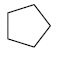d.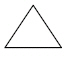b.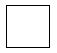e.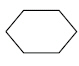c.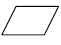3.

Which figure is not a quadrilateral?
 a.d.b.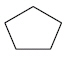e.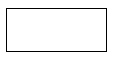c.4.

You can make a cylinder with:
 a. 3 circles d. 1 rectangle and 2 squares b. 1 circle and 1 cone e. 2 circles c. 2 circles and 1 rectangle

5.

Geometry – Symmetry and Transformations – RIT 201 – 210
Which figures are mirror images of each other?
 a.b.c.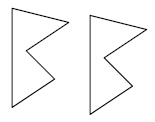d.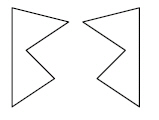e.6.

Measurement – Angle Identification & Measure – RIT 201 – 210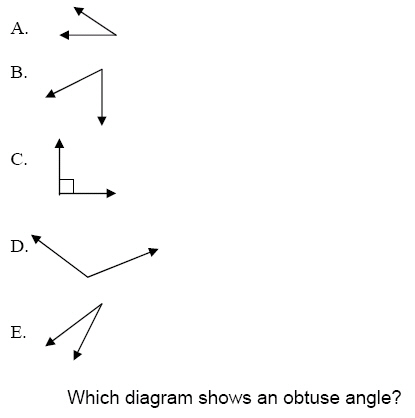a. A d. D b. B e. E c. C

7.

Measurement – Area, Perimeter, Circumference – RIT 201 – 210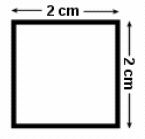What is the correct equation to find the perimeter of the square?
 a. 2cm + 2cm + 2cm = 6cm d. 2cm + 2cm = 4cm b. 2cm x 2cm = 4cm e. 2cm  2cm = 1cm c. 2cm + 2cm + 2cm + 2cm = 8cm

8.

Grass seed needed to plant 1 square meter: 1 gram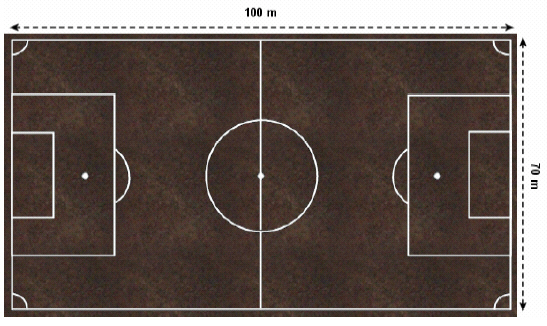If 1 gram of grass seed is needed to plant 1 square meter, how many grams of grass seed will be needed to plant the entire soccer field?
 a. 100 d. 5000 b. 70 e. 7000 c. 170

9.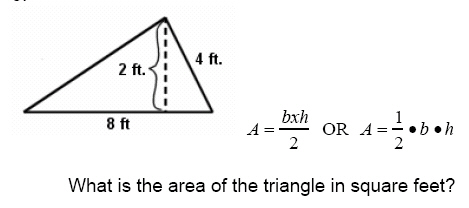a. 8 d. 10 b. 20 e. 32 c. 16

10.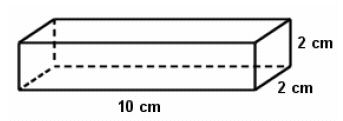What is the surface area in square centimeters?
 a. 20 d. 14 b. 88 e. 112 c. 40

11.

Measurement – Length, Weight, Volume – RIT 201 – 210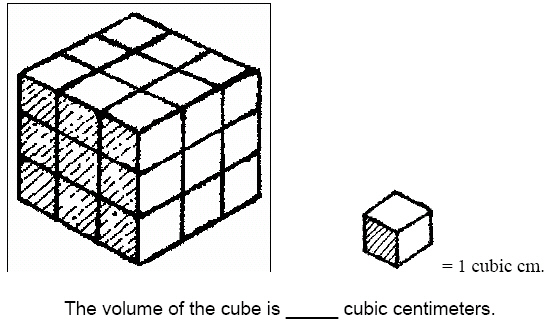a. 9 d. 27 b. 6 e. 1 c. 24

12.

947 g + 1,053 g = (Hint: 1000 g = 1 kg)
 a. 1,990 g d. 10 kg b. 894 g e. 2 kg c. 1000 g

13.

Which unit of measure would be best for measuring the area of a soccer field?
 a. meters d. inches b. centimeters e. grams c. quarts

14.

V = l • w • hWhich shows the equation to find volume in cubic feet?
 a. 4 x 4 x 4 = 64 d. 2 + 2 + 2 = 6 b. 4 x 2 x 2 = 16 e. 2 x 4 + 2 = 10 c. 4 + 2 + 2 = 8

15.

Measurement – Money – RIT 201 – 210
If Yvonne had \$5.00 and spent \$3.79, what combination of bills and coins
would equal the left-over change?
 a. 1 one-dollar bill, 1 dime, and 1 penny d. 3 one-dollar bills, 3 quarters, and 4 pennies b. 3 quarters, 4 dimes, 2 nickels, and 3 pennies e. 1 one-dollar bill, 2 dimes, and 1 penny c. 2 one-dollar bills, 7 dimes, and 9 pennies